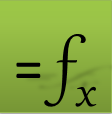# =DERIVXY(x, y, p, [options])

DERIVXY is a powerful function which employs cubic splines for estimating the derivative at an arbitrary point based on a set of (x,y) data points only.

With options, you can elect to weigh the data points, use exact or smooth least square fit, as well as specify end points slopes if known.

DERIVXY automatically sorts your data points and averages the y values if your data set contains duplicate x points.

### Required Inputs

x a vector of the points x-coordinates.

y corresponding vector of the points y-values.

p the point at which to compute the derivative.

If p is a vector of points, run DERIVXY as an array formula.

### Optional Inputs

n order of the derivative. Enter a value of 1, 2, or 3. Default is 1.

ctrl a set of key/value pairs for algorithmic control as detailed below.

Description of key/value pairs for algorithmic control

 Keys ISLOPE, ESLOPE Admissible Values real number Default Value Unconstrained Remarks If defined, the spline curve first derivative will match the supplied value.Cannot be imposed with a linear spline or with PERIOIDC option enabled.
 Key PERIODIC Admissible Values (Boolean) True or False Default Value False Remarks The y-values at the end points must be equal if PERIOIDC is set to True.ISLOPE, ESLOPE constraints are ignored
 Key SFACTOR Admissible Values (real) ≥ 0 Default Value 0 Remarks A zero value will compute an exact interpolating spline passing through the data points. A large value will compute a least-squares smooth fitting curve.The smoothing factor has a significant effect on the computed integral value.

w strictly-positive corresponding set of weights for the (x,y) data points. Default value is unity.

In this example we sample the function $f\left(x\right)=xsin\left({x}^{2}\right)+1$ then compute its derivative from the sampled data points using DERIVXY and compare the result to the analytic derivatives given by ${f}^{\text{'}}\left(x\right)=sin\left({x}^{2}\right)+2{x}^{2}cos\left({x}^{2}\right)$

### Solution

Using the formulas shown in Table 1, we generate by AutoFill the (x,y) data values and the analytic derivatives at the x-values. The numerical values are shown in Table 2 below:

 A B C 3 x_data y_data Analytic derivatives at x values 4 0 =A4*SIN(A4^2)+1 =SIN(A4^2)+2*A4^2*COS(A4^2) 5 0.25 =A5*SIN(A5^2)+1 =SIN(A5^2)+2*A5^2*COS(A5^2) 6 ⇓ Drag down to row 20
 A B C 3 x_data y_data Analytic der 4 0 1 0 5 0.25 1.015615 0.1872153 6 0.5 1.123702 0.7318602 7 0.75 1.399977 1.4849677 8 1 1.841471 1.9220756 9 1.25 2.249957 1.0258913 10 1.5 2.16711 -2.0487081 11 1.75 1.138268 -6.0268418 12 2 -0.5136 -5.9859515 13 2.25 -1.1135 2.5335616 14 2.5 0.917052 12.459939 15 2.75 3.634003 5.3043158 16 3 2.236355 -15.988226 17 3.25 -1.94996 -9.773066 18 3.5 -0.08892 22.972966 19 3.75 4.739551 3.0952707 20 4 -0.15161 -30.933007

Using DERIVXY formulas shown in Table 3, we generate by AutoFill the numerical derivatives at the x-values and compute the relative error with respect to the analytical derivatives. The numerical results are shown in Table 4 below.

Note that we have passed defined names x_data and y_data for columns A4:A20 and B4:B20 respectively. This is one way to lock the data so AutoFill ignores these arguments and increments only the third argument. Alternatively we could use a dollar sign, $, to lock the first two arguments during AutoFill using the formula =DERIVXY($A$4:$A$20,$B$4:$B\$20, A4).

 E F 3 Numerical Derivatives % Errors 4 =DERIVXY(x_data,y_data,A4) 1 5 =DERIVXY(x_data,y_data,A5) =ABS((E5-C5)/C5) ⇓ Drag down to row 20
 E F 3 Numerical Derivatives % Errors 4 -0.006256108 100.00% 5 0.189289352 1.11% 6 0.733522457 0.23% 7 1.488966928 0.27% 8 1.923837894 0.09% 9 1.015441218 1.02% 10 -2.07793704 1.43% 11 -6.043962293 0.28% 12 -5.914791221 1.19% 13 2.68187738 5.85% 14 12.35516509 0.84% 15 4.867529358 8.23% 16 -15.99364056 0.03% 17 -7.900514125 19.16% 18 19.69241868 14.28% 19 9.404971886 203.85% 20 -58.06465252 87.71%

Notice that the errors are largest near the end points of the data since the default spline fit does not constrain the derivatives at the end point. But in this example we know the exact derivatives at the end points from the analytical formula. We can improve the accuracy by supplying this data using the keys ISLOPE and ESLOPE in the optional control argument for DERIVXY. We name the range K1:L2 end_slopes and define the following Key/Value pairs for the start and end slope using the computed analytical derivative values in C4 and C20 from Table 2 above.

 K L 1 ISLOPE 0 2 ESLOPE -30.933

We regenerate the new derivatives and errors in columns H4:H20 and I4:I20 from the enhanced DERIVXY formulas in Table 5. The numerical values obtained by AutoFill are shown in Table 6 below.

 H I 3 Nummerical Derivative with end slopes % Errors 4 =DERIVXY(x_data,y_data,A4,1,end_slopes) 1 5 =DERIVXY(x_data,y_data,A5,1,end_slopes) =ABS((H5-C5)/C5) ⇓ Drag down to row 20
 H I 3 Num. Der. with end slopes % Errors 4 0 0.00% 5 0.187612966 0.21% 6 0.733971891 0.29% 7 1.488845578 0.26% 8 1.923873859 0.09% 9 1.015418708 1.02% 10 -2.077882964 1.42% 11 -6.044156087 0.29% 12 -5.91407012 1.20% 13 2.679186771 5.75% 14 12.36520643 0.76% 15 4.830054635 8.94% 16 -15.853783 0.84% 17 -8.422469647 13.82% 18 21.64038321 5.80% 19 2.135069296 31.02% 20 -30.93300669 0.00%

Notice the improvement in the relative error with the defined end points slopes. Keep in mind that we are working with a relatively small sample size. To obtain any further improvement would require finer sampling.

DERIVXY computes the derivative by fitting a cubic spline model to the data.

Question or Comment? Email us:
support @ excel-works.comExceLab: Transforming Excel into a Calculus Power House

ExceLab functions and methods are protected by USA Patents 10628634, 10114812, 9892108 and 9286286.